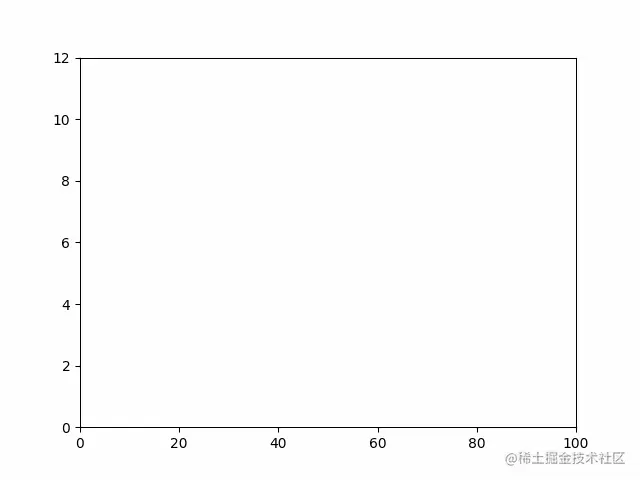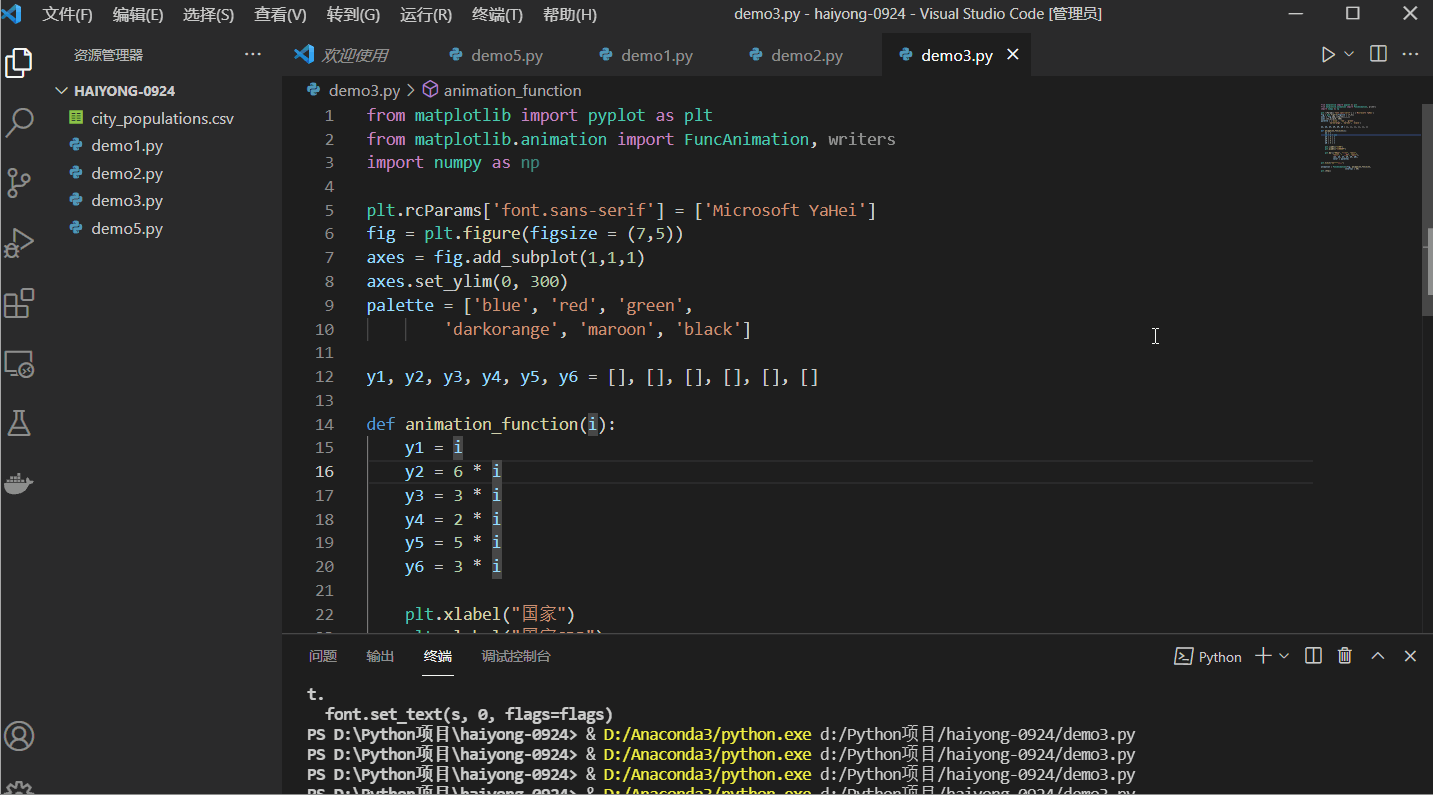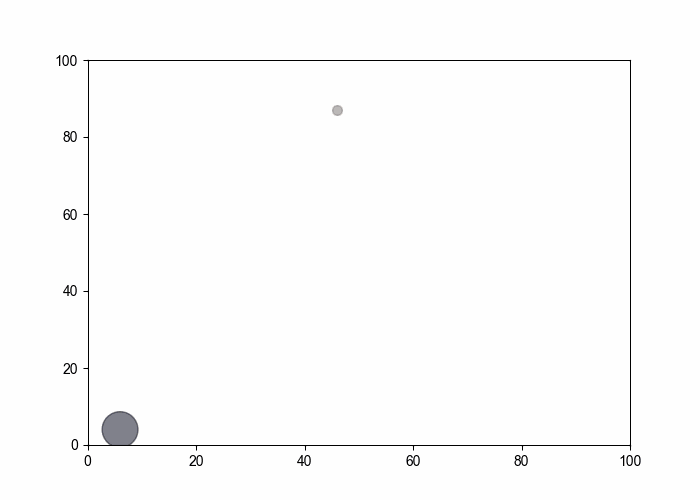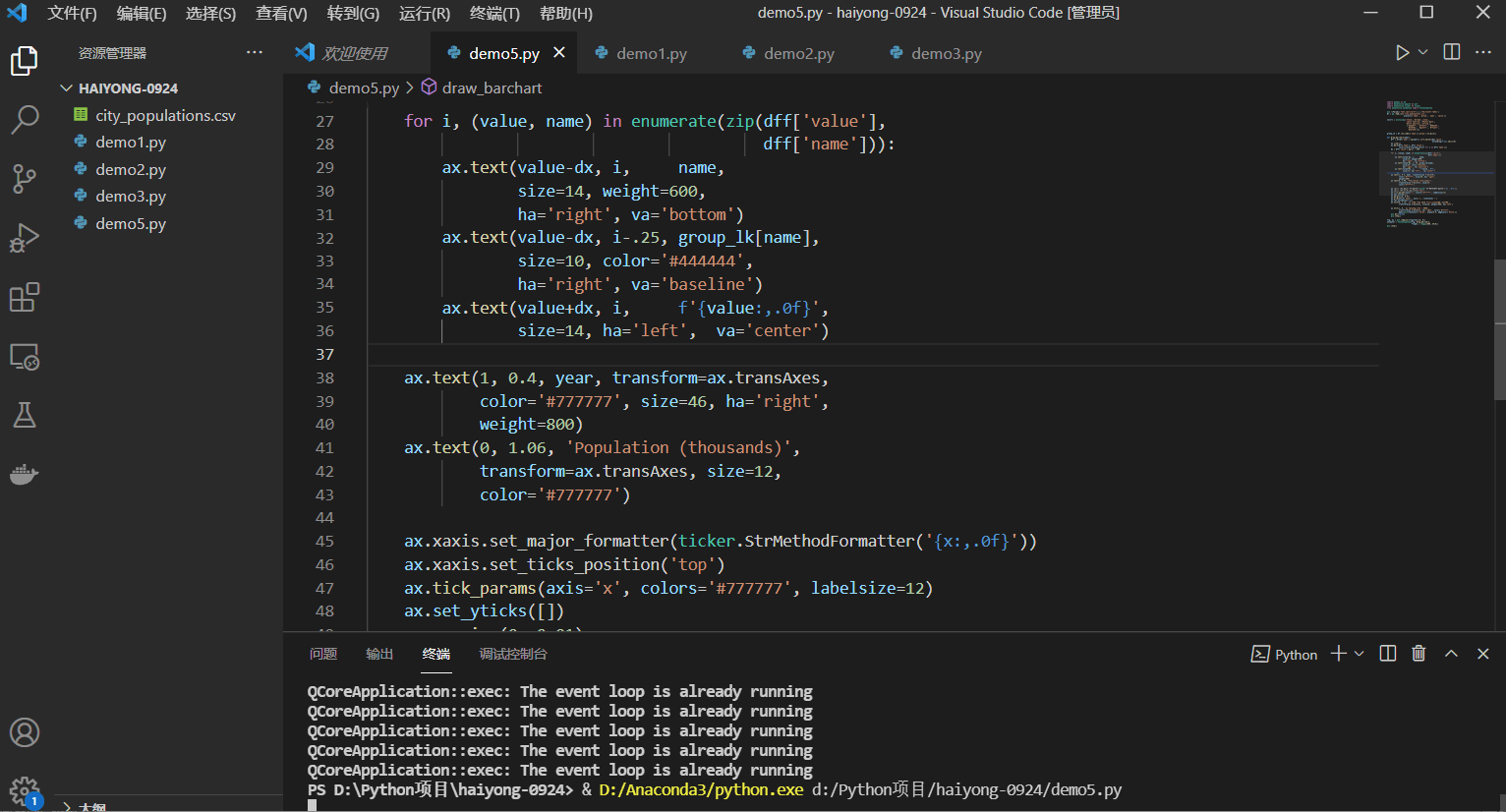# [introduction to Python project] create bar chart animation in Python

2022-01-29 10:38:47 Haiyong

This article has participated in 「 Digging force Star Program 」, Win a creative gift bag , Challenge creation incentive fund .

【 Comment lottery 】「 Welcome to discuss in the comment area , Nuggets officials will be in Digging force Star Program After the event , Draw... In the comment area 100 Around the Nuggets , For details of the lucky draw, see the activity article 」

Animation is a good way to make visualization more attractive and user attractive . It helps us show data visualization in a meaningful way .Python Help us use existing powerful Python Library to create animation visualization .Matplotlib Is a very popular data visualization Library , It is usually used for graphical representation of data and animation using built-in functions .

Use Matplotlib There are two ways to create animation ：

• Use pause() function
• Use FuncAnimation() function

## Method 1 ： Use pause() function

On hold （） Of matplotlib Library pyplot The module is functionally used to pause the interval seconds mentioned for the parameter . Consider the following example , We will use matplotlib Create a simple linear graph and display animation in it ：

establish 2 An array X and Y, And store from 1 To 100 Value . Use plot() Function to draw X and Y. Add at appropriate intervals pause() function Run the program , You will see the animation .

Python

``````from matplotlib import pyplot as plt

x = []
y = []

for i in range(100):
x.append(i)
y.append(i)

#  mention  x  and  y  Limit to define its scope
plt.xlim(0, 100)
plt.ylim(0, 100)

#  Drawing graphics
plt.plot(x, y, color = 'green')
plt.pause(0.01)

plt.show()
Copy code ``````

Output ：Again , You can also use pause() Function to create animation in various drawings .

## Method 2 ： Use FuncAnimation() function

This FuncAnimation() Functions do not create their own animation , Instead, we create animation from a series of graphics we pass .

grammar : FuncAnimation(figure, animation_function, frames=None, init_func=None, fargs=None, save_count=None, *, cache_frame_data=True, **kwargs)

Now you can use FuncAnimation Function to animate multiple types ：

### Linear graph animation ：

In this case , We will create a simple linear graph , It displays an animation of a line . Again , Use FuncAnimation, We can create many types of animated visual representations . We just need to define our animation in a function , Then pass it to with the appropriate parameters FuncAnimation.

Python

``````from matplotlib import pyplot as plt
from matplotlib.animation import FuncAnimation
import numpy as np

x = []
y = []

figure, ax = plt.subplots()

#  Set up  x  and  y  Axis limitation
ax.set_xlim(0, 100)
ax.set_ylim(0, 12)

#  Draw a single graphic
line,  = ax.plot(0, 0)

def animation_function(i):
x.append(i * 15)
y.append(i)

line.set_xdata(x)
line.set_ydata(y)
return line,

animation = FuncAnimation(figure,
func = animation_function,
frames = np.arange(0, 10, 0.1),
interval = 10)
plt.show()
Copy code ``````

Output ：## Python Bar chart in catch-up animation

In this example , We will create a simple bar graph animation , It displays the animation for each bar .

Python

``````from matplotlib import pyplot as plt
from matplotlib.animation import FuncAnimation, writers
import numpy as np

plt.rcParams['font.sans-serif'] = ['Microsoft YaHei']
fig = plt.figure(figsize = (7,5))
axes.set_ylim(0, 300)
palette = ['blue', 'red', 'green',
'darkorange', 'maroon', 'black']

y1, y2, y3, y4, y5, y6 = [], [], [], [], [], []

def animation_function(i):
y1 = i
y2 = 6 * i
y3 = 3 * i
y4 = 2 * i
y5 = 5 * i
y6 = 3 * i

plt.xlabel(" Country ")
plt.ylabel(" Country GDP")

plt.bar([" India ", " China ", " Germany ",
" The United States ", " Canada ", " The British "],
[y1, y2, y3, y4, y5, y6],
color = palette)

plt.title(" Bar graph animation ")

animation = FuncAnimation(fig, animation_function,
interval = 50)
plt.show()
Copy code ``````

Output ：## Python Scatter animation in ：

In this case , We will use random functions in python Animated scatter in . We're going to traverse animation_func And draw during iteration x and y Random value of axis .

``````from matplotlib import pyplot as plt
from matplotlib.animation import FuncAnimation
import random
import numpy as np

x = []
y = []
colors = []
fig = plt.figure(figsize=(7,5))

def animation_func(i):
x.append(random.randint(0,100))
y.append(random.randint(0,100))
colors.append(np.random.rand(1))
area = random.randint(0,30) * random.randint(0,30)
plt.xlim(0,100)
plt.ylim(0,100)
plt.scatter(x, y, c = colors, s = area, alpha = 0.5)

animation = FuncAnimation(fig, animation_func,
interval = 100)
plt.show()
Copy code ``````

Output ：## * The bar chart catches up with the horizontal movement ：

ad locum , We will use the highest population in the city dataset to draw a bar graph contest . Different cities have different bar charts , The bar graph will start from 1990 Year to 2018 Annual iteration . I chose the country with the highest city from the most populous data set . The required data sets can be downloaded here ：city_populations

Python

``````import pandas as pd
import matplotlib.pyplot as plt
import matplotlib.ticker as ticker
from matplotlib.animation import FuncAnimation

plt.rcParams['font.sans-serif'] = ['Microsoft YaHei']
usecols=['name', 'group', 'year', 'value'])

colors = dict(zip(['India','Europe','Asia',
'Latin America','Middle East',
'North America','Africa'],
'#e48381', '#aafbff', '#f7bb5f',
'#eafb50']))

group_lk = df.set_index('name')['group'].to_dict()

def draw_barchart(year):
dff = df[df['year'].eq(year)].sort_values(by='value',
ascending=True).tail(10)
ax.clear()
ax.barh(dff['name'], dff['value'],
color=[colors[group_lk[x]] for x in dff['name']])
dx = dff['value'].max() / 200

for i, (value, name) in enumerate(zip(dff['value'],
dff['name'])):
ax.text(value-dx, i,     name,
size=14, weight=600,
ha='right', va='bottom')
ax.text(value-dx, i-.25, group_lk[name],
size=10, color='#444444',
ha='right', va='baseline')
ax.text(value+dx, i,     f'{value:,.0f}',
size=14, ha='left',  va='center')

ax.text(1, 0.4, year, transform=ax.transAxes,
color='#777777', size=46, ha='right',
weight=800)
ax.text(0, 1.06, 'Population (thousands)',
transform=ax.transAxes, size=12,
color='#777777')

ax.xaxis.set_major_formatter(ticker.StrMethodFormatter('{x:,.0f}'))
ax.xaxis.set_ticks_position('top')
ax.tick_params(axis='x', colors='#777777', labelsize=12)
ax.set_yticks([])
ax.margins(0, 0.01)
ax.grid(which='major', axis='x', linestyle='-')
ax.set_axisbelow(True)
ax.text(0, 1.12, ' from  1500  Year to  2018  The most populous city in the world in ',
transform=ax.transAxes, size=24, weight=600, ha='left')

ax.text(1, 0, 'by haiyong.site |  Hai Yong ',
transform=ax.transAxes, ha='right', color='#777777',
bbox=dict(facecolor='white', alpha=0.8, edgecolor='white'))
plt.box(False)
plt.show()

fig, ax = plt.subplots(figsize=(15, 8))
animator = FuncAnimation(fig, draw_barchart,
frames = range(1990, 2019))
plt.show()
Copy code ``````

Output ：## wuhu ! take off !

I've been writing a technology blog for a long time , And mainly through the Nuggets , This is my article python Getting started project tutorial . I like to share technology and happiness through articles . You can visit my blog ： juejin.cn/user/204034… To learn more . I hope you will like ！

You are welcome to put forward your opinions and suggestions in the comment area ！ Nuggets officials will be in Digging force Star Program After the event , Draw... In the comment area 100 Around the Nuggets , For details of the lucky draw, see the activity article 」

If you really learn something new from this article , Like it , Collect it and share it with your friends . Last , Don't forget or support .